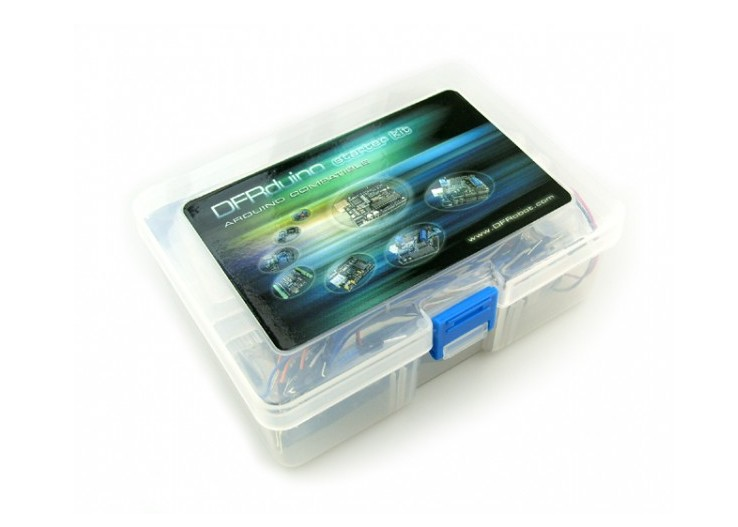## Introduction

This beginner kit is just designed for newbie in Arduino. It gives you a basic introduction to the Arduino world. Starts from basic LED control to more advanced IR remote control. You can enjoy lots of fun from it.

## What is included?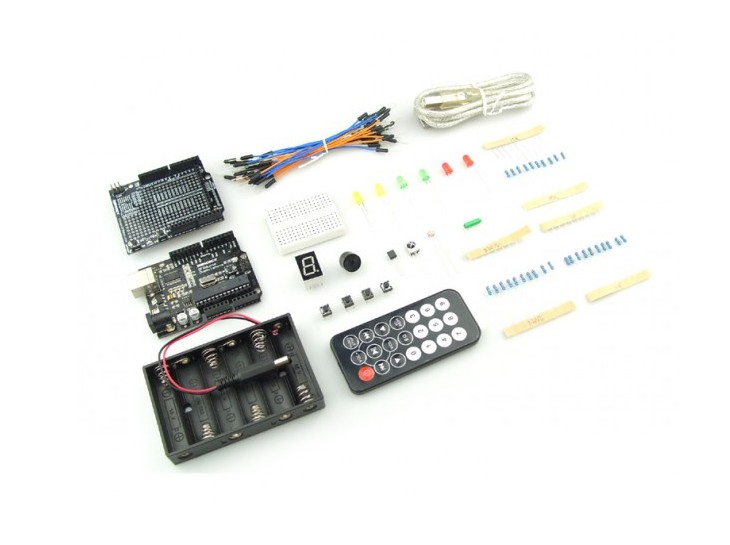## LED Control Tutorial

### Connection Diagram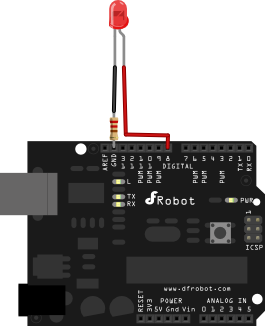``````
//LED 1 Sample Code
//For Arduino Start kit
//Compatible with all Arduino version
//Last updated 2010-12-1
//www.dfrobot.com

int ledPin=8; //Connect LED to Digital Pin 8
void setup()
{
pinMode(ledPin,OUTPUT);//Set ledPin to output mode
}
void loop()
{
digitalWrite(ledPin,HIGH); //Pull ledPin to HIGH which is 5V
delay(1000); //set 1 second delay (1000 =  1 second)
digitalWrite(ledPin,LOW); //Set ledPin to low which is 0V
delay(1000); //set 1 second delay (1000 =  1 second)
}``````

## LED 2 Control Tutorial

### Connection Diagram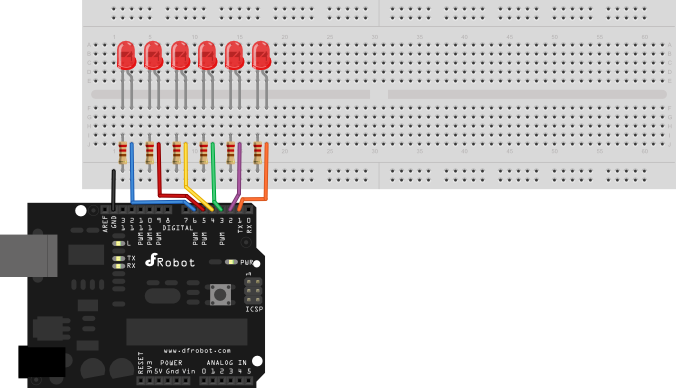``````
//LED 2 Sample Code
//For Arduino Start kit
//Compatible with all Arduino version
//Last updated 2010-12-1
//www.dfrobot.com

int Led1 = 1;
int Led2 = 2;
int Led3 = 3;
int Led4 = 4;
int Led5 = 5;
int Led6 = 6;

void style_1(void)
{
unsigned char j;
for(j=1;j<=6;j  )
{
digitalWrite(j,HIGH);
delay(200);//
}
for(j=6;j>=1;j--)
{
digitalWrite(j,LOW);
delay(200);
}
}

void flash(void)
{
unsigned char j,k;
for(k=0;k<=1;k  )
{
for(j=1;j<=6;j  )
digitalWrite(j,HIGH);
delay(200);
for(j=1;j<=6;j  )
digitalWrite(j,LOW);
delay(200);
}
}

void style_2(void)
{
unsigned char j,k;
k=1;
for(j=3;j>=1;j--)
{
digitalWrite(j,HIGH);
digitalWrite(j k,HIGH);
delay(400);
k  =2;
}
k=5;
for(j=1;j<=3;j  )
{
digitalWrite(j,LOW);
digitalWrite(j k,LOW);
delay(400);
k -=2;
}
}

void style_3(void)
{
unsigned char j,k;
k=5;
for(j=1;j<=3;j  )
{
digitalWrite(j,HIGH);
digitalWrite(j k,HIGH);
delay(400);
digitalWrite(j,LOW);
digitalWrite(j k,LOW);
k -=2;
}
k=3;
for(j=2;j>=1;j--)
{
digitalWrite(j,HIGH);
digitalWrite(j k,HIGH);
delay(400);
digitalWrite(j,LOW);
digitalWrite(j k,LOW);
k  =2;
}
}
void setup()
{
unsigned char i;
for(i=1;i<=6;i  )
pinMode(i,OUTPUT);
}
void loop()
{
style_1();
flash();
style_2();
flash();
style_3();
flash();
}``````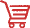Get *DFRduino Beginner Kit For Arduino SKU DFR0100 * from DFRobot Store or DFRobot Distributor.